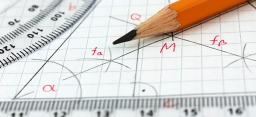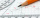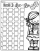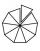# Calculate 20

Calculate the sum of 1/5 of a right angle and 3/4 of a right angle and 3/4 of a straight angle

s =  220.5 °

### Step-by-step explanation:Did you find an error or inaccuracy? Feel free to write us. Thank you!Tips to related online calculators
Need help to calculate sum, simplify or multiply fractions? Try our fraction calculator.

## Related math problems and questions:

• A straight angleA straight angle measures s, so you can subtract or add 85 from What are the measure of there angles?
• The sum 12The sum of 3 mixed numbers is 20 13/15. two of the numbers are 6 1/3 and 7 5/6. what is the third number?
• Game 27Susan wanted to play the game. In the beginning, the first says a number from 1 to 8. Then the second player adds a number from 1 to 5 and tells the sum. Again, the Susan adds a number from 1-5 and say sum and etc. . . The winner must say the number 27. W
• Fractions and mixed numerals(a) Convert the following mixed numbers to improper fractions. i. 3 5/8 ii. 7 7/6 (b) Convert the following improper fraction to mixed number. i. 13/4 ii. 78/5 (c) Simplify these fractions to their lowest terms. i. 36/42 ii. 27/45 2. evaluate following ex
• Two numbers 11The sum of two rational numbers is (-2). If one of them is 3/5, find the other.
• Sum of 18Sum of two fractions is 4 3/7. If one of the fractions is 2 1/5 find the other one .
• Two numbersThe sum of two numbers is 1. Identify this two numbers if you know that the half of first is equal to the third of second number.
• Circular arcCalculate the length of the circular arc if the diameter d = 20cm and the angle alpha = 142 °
• The sum 10The sum of three decimals are 938.629. Two of them are 456.54 and 392.69. Find the third one.
• NumbersDetermine the number of all positive integers less than 4183444 if each is divisible by 29, 7, 17. What is its sum?
• Irrigation sprinklerThe irrigation sprinkler can twist with an angle of 320° and has a reach of 12 meters. Which area can irrigate?To three-eighths of one third, we add five quarters of one half and multiply the sum by four. How much will we get?The sum of the first 10 members of the arithmetic sequence is 120. What will be the sum if the difference is reduced by 2?Find 2/3 of the sum's ratio and the product of all prime divisors of the number 120.Sum of interiol angles of 9-gon is:The sum of three numbers from which second number is 20% smaller than the first number and the third number is 25% smaller than the second number is 96. Determine this numbers.On each wall of a regular octahedron is written one of the numbers 1, 2, 3, 4, 5, 6, 7 and 8, wherein on different sides are different numbers. For each wall John make the sum of the numbers written of three adjacent walls. Thus got eight sums, which also